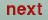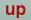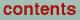## Machine constants

The following machine dependent constants are used in the TB-LMTO prog. They are supplied in functions in extens.f:

dmach(1)=1.d-99 [ smallest double precision number]

dmach(2)=1.d+99 [ largest double precision number]

dmach(3)=1.d-15 [ smallest number which added to 1.0 gives something different from 1.0 ]

imach(2)=6 [ standard output channel ]

imach(4)=6 [ standard error channel ]

imach(6)=4 [ no. characters per integer ]

imach(17)=1 [ no. integer words per real word ]

imach(18)=2 [ no. integer words per double precision word ]O. Jepsen
Thu Oct 12 14:48:45 MESZ 2000